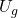## A 0.25 kg book falls off a 2 m shelf on to a 0.5 m chair. What was the change in GPE?

Question

A 0.25 kg book falls off a 2 m shelf on to a 0.5 m chair. What was the change in GPE?

in progress 0
5 months 2021-08-30T07:08:44+00:00 1 Answers 4 views 0

1. The equation of GPE is mgH, where m is mass, g is gravitational acceleration, and H is the height.

If we’re solving for the change in GPE, then:= mg∆H

Input our given values for m and g:= 0.25 * 9.80 * ∆H

The book falls from 2 meters high to 0.5 meters high, so:= 0.25 * 9.80 * (2.0 – 0.5)= 0.25 * 9.80 * 1.5= 3.675 (J)= 3.7 (J)